# Heaviside Function facts for kids

Kids Encyclopedia Facts

The Heaviside function, often written as H(x), is a non-continuous function whose value is zero for a negative input and one for a positive input.

The function is used in the mathematics of control theory to represent a signal that switches on at a specified time, and which stays switched on indefinitely. It was named after the Englishman Oliver Heaviside.

The Heaviside function is the integral of the Dirac delta function: H′(x) = δ(x). This is sometimes written as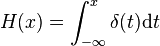$H(x) = \int_{-\infty}^x { \delta(t)} \mathrm{d}t$

## Discrete form

We can also define an alternative form of the Heaviside step function as a function of a discrete variable n: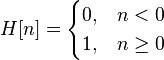$H[n]=\begin{cases} 0, & n < 0 \\ 1, & n \ge 0 \end{cases}$

where n is an integer.

Or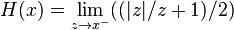$H(x) = \lim_{z \rightarrow x^-} ((|z| / z + 1) / 2)$

The discrete-time unit impulse is the first difference of the discrete-time step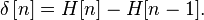$\delta\left[ n \right] = H[n] - H[n-1].$

This function is the cumulative summation of the Kronecker delta: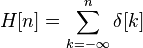$H[n] = \sum_{k=-\infty}^{n} \delta[k] \,$

where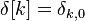$\delta[k] = \delta_{k,0} \,$

is the discrete unit impulse function.

## Representations

Often an integral representation of the Heaviside step function is useful: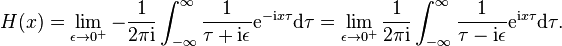$H(x)=\lim_{ \epsilon \to 0^+} -{1\over 2\pi \mathrm{i}}\int_{-\infty}^\infty {1 \over \tau+\mathrm{i}\epsilon} \mathrm{e}^{-\mathrm{i} x \tau} \mathrm{d}\tau =\lim_{ \epsilon \to 0^+} {1\over 2\pi \mathrm{i}}\int_{-\infty}^\infty {1 \over \tau-\mathrm{i}\epsilon} \mathrm{e}^{\mathrm{i} x \tau} \mathrm{d}\tau.$

## H(0)

The value of the function at 0 can be defined as H(0) = 0, H(0) = ½ or H(0) = 1. In particular: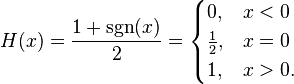$H(x) = \frac{1+\sgn(x)}{2} = \begin{cases} 0, & x < 0 \\ \frac{1}{2}, & x = 0 \\ 1, & x > 0. \end{cases}$

## Related pagesHeaviside Function Facts for Kids. Kiddle Encyclopedia.